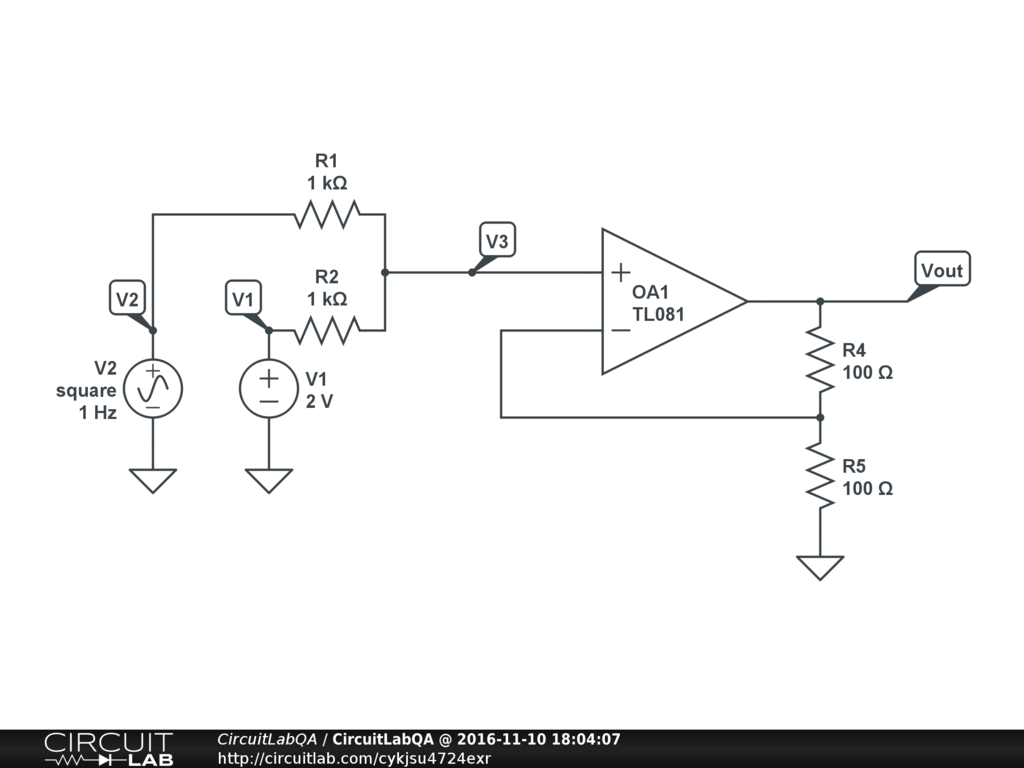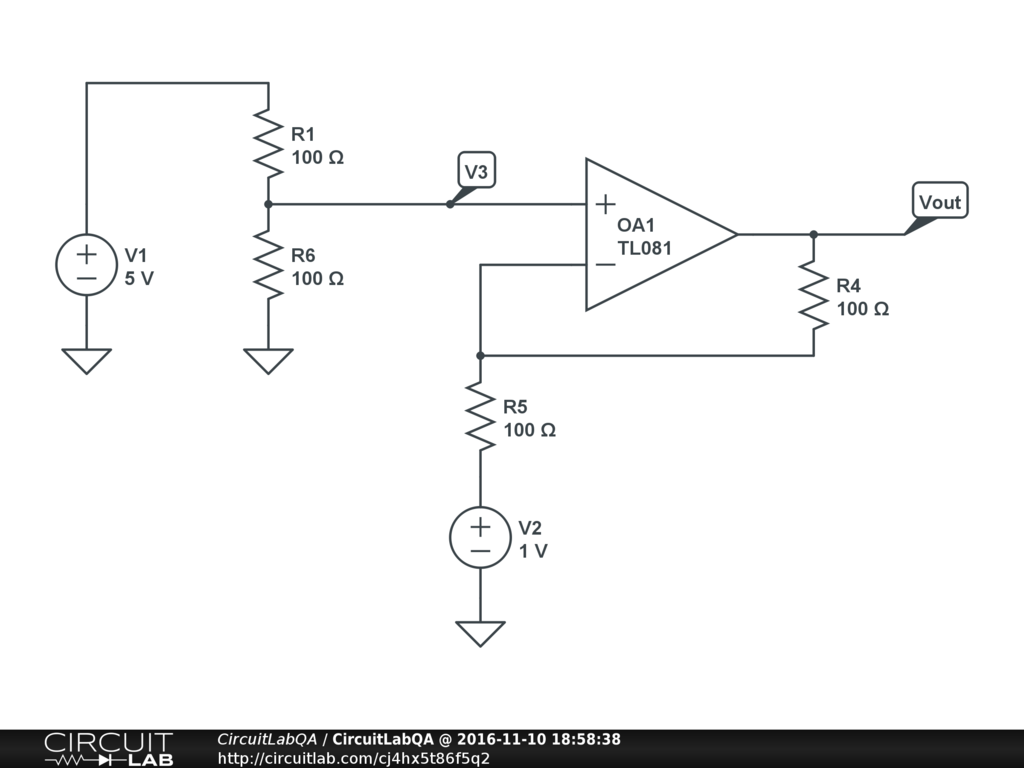## Getting the sum and difference of two signals with op amps? SOLVED

 I have two signals, one is a baseline voltage $V_{1}$ and the other is a difference voltage $V_{2}$. From these I want to produce two signals, $V_{1}$ + $V_{2}$ and $V_{1}$ + $V_{2}$. Is this possible? by jkary November 10, 2016

 This is totally possible. Lets take the circuits one by one, looking this adder circuit first.Notice how its just a voltage divider between the two inputs, plugged into an amplifier with a gain of 2. Go ahead and drop into the simulator and probe around the circuit. An interesting one to look at is the DC Sweep, that sweeps the voltage of V2 from 0-5 linearly, and you can verify that $V_{out}$ is always $V_{1} + V_{2}$ Now take a look at the difference circuit below that calculates $V_{out} = V_{1} - V_{2}$You can use unity gain buffers to hook these two up to your two inputs. ACCEPTED +2 votes by agiustino45 November 10, 2016

CircuitLab's Q&A site is a FREE questions and answers forum for electronics and electrical engineering students, hobbyists, and professionals.

We encourage you to use our built-in schematic & simulation software to add more detail to your questions and answers.

Acceptable Questions:

• Concept or theory questions
• Practical engineering questions
• “Homework” questions
• Software/hardware intersection
• Best practices
• Design choices & component selection
• Troubleshooting

Unacceptable Questions:

• Non-English language content
• Non-question discussion
• Non-electronics questions
• Vendor-specific topics
• Pure software questions
• CircuitLab software support

Please respect that there are both seasoned experts and total newbies here: please be nice, be constructive, and be specific!